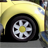# Reclassify by class

1328
5
02-04-2013 07:24 AMOccasional Contributor
Hello,

I'm writing a python script in ArcGIS 10.1 in which I want to reclassify a raster as follows:

First, classify into three classes using the standard deviation method. Second, reclassify the raster's values as like this:

1st class = 1
2nd class = 2
3rd class = 3

There are two problems here, or at least I think there are two problems. The first is that there doesn't appear to be a way in ArcPy to specify the number of classes or the classification method. I tried saving a reference .lyr where a 3 class standard deviation symbology is set and then using arcpy.mapping.UpdateLayer but this takes the exact class values and applies them rather than taking just the classification method and number of classes.

The second problem is how to reclassify the cells in each class to values of 1,2,3 respectively. I don't care what the values of the cells in those classes are and in fact I don't know what they are. Because this script will be used many different times with many different input rasters I can't have a hard coded Remap table; the input raster values will be different each time. The Remap object as described here will reclassify by value or range; this is not what I want to do. I want to say, "take the first class and set those cells = 1; take the second class and set those cells = 2; take the third class and set those cells = 3". I will then convert the result to polygon so that I can use Select by Location on another dataset: "select features where value = 1; select features from another dataset which are contained by the first selection, and do some stuff to them".

I'd really appreciate any ideas you have that could help in either applying a new 3 class standard deviation classification to a raster or in reclassifying a classified raster as described above.

Thanks,
Ryan
Tags (3)
1 Solution

Accepted SolutionsOccasional Contributor
For the first problem the answer is here. Once I've applied the symbology from the saved .lyr file I have to use the RasterClassifiedSymbology.reclassify() method to force the classification to update itself using values from my dataset.

Cheers,
Ryan
5 RepliesOccasional Contributor
For the first problem the answer is here. Once I've applied the symbology from the saved .lyr file I have to use the RasterClassifiedSymbology.reclassify() method to force the classification to update itself using values from my dataset.

Cheers,
RyanOccasional Contributor
For the first problems the answer is here. Once I've applied the symbology from the saved .lyr file I have to use the RasterClassifiedSymbology.reclassify() method to force the classification to update itself using values from my dataset.

Cheers,
Ryan

Awesome. Thanks Ryan, you're the best! Now I just need a solution for the second part - reclassifying by relative class (i.e. first class, second class, third class) instead of by cell value.

RyanOccasional Contributor
RasterClassifiedSymbology solves the second problem as well. It provides access to the classBreakValues and numClasses properties so I can make sure I have 3 classes and then construct a Remap object by reading the classBreakValues.

This Ryan guy is a genius!

RyanNew Contributor III
Hello,
I have what I think is a similar question or perhaps I can get pointed in the right direction.
In model builder or python script that I can then import into model builder, I want to be able to have the user specify slope percentage breaking points. Would it be possible to do with this tool?  In model builder I can calculate the percent rise but instead of using a predefined remap table I would like the user to specify the breaking points. Or maybe I could created a field with pre existing breaking points and have the user select from those options and then convert to vector?
Could I save my classifications as a layer file and have the user pick from a layer file so the slopes will reclassify and then export?  Basically I as thinking of having maybe three option for slope breaking points. (0-10, 10-20 >20; 0-15, 15-30, >30; 0-20, 20-40, >40)
Could this be configured.
I am using ArcGIS 10.0byMVP Esteemed Contributor
I'm writing a python script in ArcGIS 10.1 in which I want to reclassify a raster as follows:

First, classify into three classes using the standard deviation method. Second, reclassify the raster's values as like this:

1st class = 1
2nd class = 2
3rd class = 3

There are two problems here, or at least I think there are two problems. The first is that there doesn't appear to be a way in ArcPy to specify the number of classes or the classification method. I tried saving a reference .lyr where a 3 class standard deviation symbology is set and then using arcpy.mapping.UpdateLayer but this takes the exact class values and applies them rather than taking just the classification method and number of classes.

The second problem is how to reclassify the cells in each class to values of 1,2,3 respectively.

You can do this with Python map algebra (if you have Spatial Analyst available):

```from arcpy.sa import *
ras = Raster("valueraster")
classes = 3
stdevs = 1
base = 1 # output raster is 1,2,3,...
newmin = ras.mean - (stdevs * ras.standardDeviation)
newmax = ras.mean + (stdevs * ras.standardDeviation)
# set slicing min and max
temp = Con(ras < newmin, newmin, Con(ras > newmax, newmax, ras))
newras = Slice(temp,"EQUAL_INTERVAL, classes, base)
```

If you need the break values, you can get them using ZonalStatisticsAsTable using newras as your zone raster and "valueraster" for the values.

This method is probably more accurate than using arcpy.mapping if your data are highly skewed, because the layer statistics may be developed from a smaller sample of the data than your actual raster statistics.